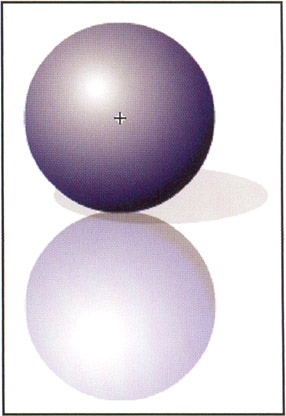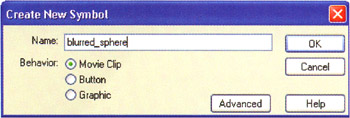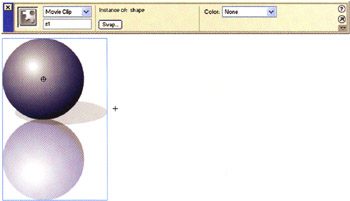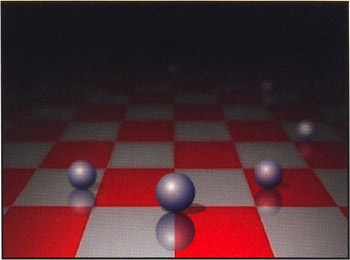In this example we'll again examine the concept of approaching the plane of focus, but this time we'll come at it from a programmatic angle and use ActionScript to create some neat pseudo-3D effects. The source file for this example is focus3.fla .

1. Select File > New to create a new movie, and then choose Modify > Document to edit the movie properties. Set the Dimensions to 400x300 and the Frame Rate to 30 fps.

2. In this movie, you will animate a series of balls. Your ball is made up of three circles. The first circle uses a gradient so that it looks like light is striking it from one side (if you haven't already done so, it's worth taking a look at Chapter 2, where we examine the use of shading and highlighting to create faux-3D effects). The second circle is a duplicate of the first that has been mirrored vertically. The third circle is simply a black circle that represents a shadow. Set the alpha on the second and third balls so that the background will show through. Save this graphic as a movie clip named sphere .3. Now create another movie clip. On the main timeline, select Insert > New Symbol (CTRL/CMD+F8) and name the new symbol blurred_sphere .4. Drag an instance of sphere from the Library (F11) onto the stage of blurred_sphere and name the instance s1 .5. On frame 1 of the blurred_sphere movie clip, add the following ActionScript:

for (i=2; i<=4; i++) {   s1.duplicateMovieClip("s"+i, i);   }   ar = s1._width/s1._height;   function set_blur(blur) {   o = (blur/100)*4;   for (i=1; i<=4; i++) {   eval("s"+i)._x = o*Math.cos(i*1.57);   eval("s"+i)._y = o*Math.sin(i*1.57);   eval("s"+i)._alpha = 10+(50*(100-blur)/100);   eval("s"+i)._width = ar*(20+(70*(100-blur)/100));   eval("s"+i)._height = (20+(70*(100-blur)/100));   }   }

The first line in this code segment creates three more copies of the sphere movie clip that will be named s2 , s3 , and s4 . On the second line, we note the width to height ratio of the sphere movie clip in the variable ar . We then define the set_blur function, which performs three important operations on each of the four sphere instances. First, set_blur adjusts the position of each sphere so that as the blur variable increases , each instance moves farther from the center of the movie. Second, set_blur adjusts the _alpha so that as the blur variable increases, the _alpha decreases. And third, set_blur adjusts the _width and the _height so that the size is also dependent on the blur value.

1. Return to the main timeline. At this point, you need to create a surface that your spheres can glide across. The surface should be sharp in the foreground and indistinct in the distance. In the demonstration file, focus3.fla , we've created an image in Photoshop by performing a perspective transformation on a checkerboard pattern and then adding a linear gradient from the top of the image to the bottom, which we used as a selection mask for the Gaussian Blur filter. This has the effect of blurring the top of the image more than the bottom. We then applied a black-to-transparent gradient from the top of the image to the bottom to make the background seem darker .

2. Now drag an instance of the blurred_sphere symbol from the Library window onto the stage, and name this instance bs1 .

3. On frame 1 of the top layer, add the following ActionScript:

t = 0;   n = 6;   for (i=2; i<=n; i++) {   bs1.duplicateMovieClip("bs"+i, i);   }   this.onEnterFrame = function() {  // increment the position along the path  t += (.1745/4);  // Keep values in the range 0 to 2*pi  if (t>6.283) {   t -= 6.283;   }  // Iterate over all n (6) balls  for (i=1; i<=n; i++) {  // Reference a particular ball bs1 .. m6  bs = eval("bs"+i);  // offset each ball by .785 radians or 45 degrees  u = t+i*.785;  // Calculate how much blur to apply based on position  blur = Math.abs(Math.sin((u/2)-.785)*100);   bs.set_blur(blur);  // The width of the ellipse is 150*2 pixels  bs._x = 150*Math.cos(u)+200;  // The height of the ellipse is 70*2 pixels  bs._y = 70*Math.sin(u)+150;   }   };

The position along the elliptical path is determined by the variable t , where t = 0 corresponds to the beginning of the path and t= 6.283 (or approximately 2 times ) corresponds to the point where the ball has come full circle. Thus, by changing how much we increment t , we can change the speed at which the balls travel. Within the onEnterFrame function, we use a second variable, u , which has an offset dependent on the index of the sphere. So, for example, m2 will arrive at the origin slightly after m1 . In this way, we can increment a single variable t that can then be used to calculate the position of all of the spheres.

1. Test the movie. The final animation is swift and convincing. Spheres glide in an elliptical path in and out of focus. The shadows and reflections help establish the placement of the spheres on the floor, and the focus truly establishes the depth of each sphere in the scene.Flash 3D Cheats Most Wanted
ISBN: 1590592212
EAN: N/A
Year: 2002
Pages: 97